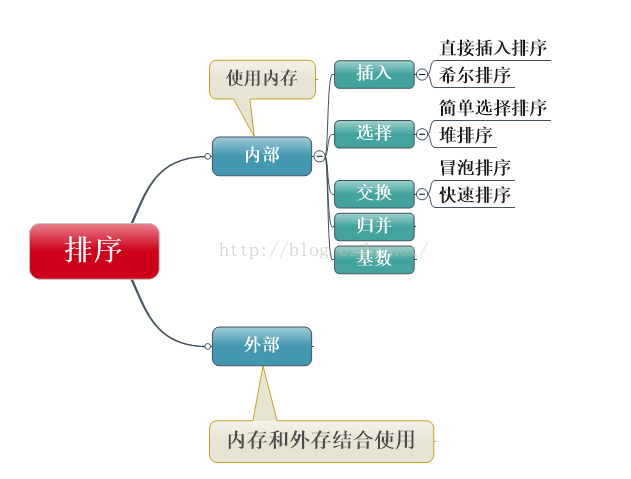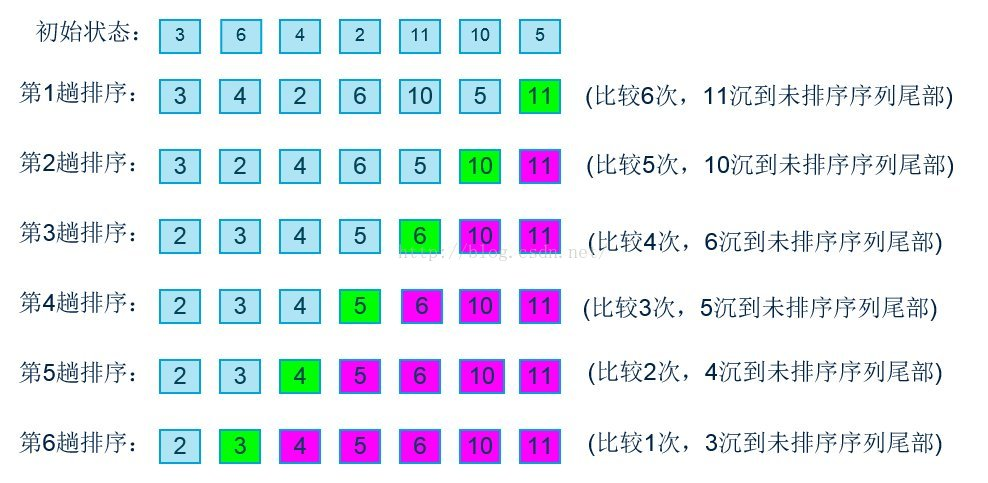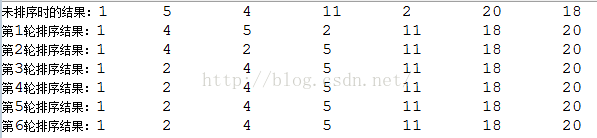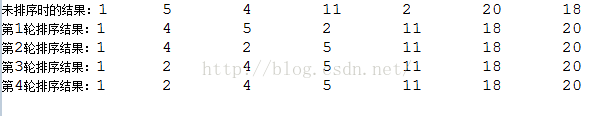# Java冒泡排序

1508℃

### Java冒泡排序

#### 排序算法概述java实现

`````` public class Sort{

private int [] array;
public Sort(int [] array){
this.array = array;
}

//按顺序打印数组中的元素
public void display(){
for(int i=0;i<array.length;i++){
System.out.print(array[i]+"\t");
}
System.out.println();
}

//冒泡排序
public void bubbleSort(){
int temp;
int len = array.length;
for(int i=0;i<len-1;i++){ //外层循环：每循环一次就确定了一个相对最大元素
for(int j=1;j<len-i;j++){ //内层循环：有i个元素已经排好，根据i确定本次的比较次数
if(array[j-1]>array[j]){ //如果前一位大于后一位，交换位置
temp = array[j-1];
array[j-1] = array[j];
array[j] = temp;
}
}
System.out.print("第"+(i+1)+"轮排序结果：");
display();
}
}
}  ``````

`````` public static void main(String[] args) {
int [] a = {1,5,4,11,2,20,18};
Sort sort = new Sort(a);
System.out.print("未排序时的结果：");
sort.display();
sort.bubbleSort();
}  ``````(N-1)+ (N-2)+ (N-3)+ …1=N*(N-1)/2

``````   //冒泡排序改进1
public void bubbleSort_improvement_1(){
int temp;
int len = array.length;

for(int i=0;i<len-1;i++){
boolean exchange = false; //设置交换变量
for(int j=1;j<len-i;j++){
if(array[j-1]>array[j]){ //如果前一位大于后一位，交换位置
temp = array[j-1];
array[j-1] = array[j];
array[j] = temp;

if(!exchange) exchange =true; //发生了交换操作
}
}
System.out.print("第"+(i+1)+"轮排序结果：");
display();
if(!exchange) break; //如果上一轮没有发生交换数据，证明已经是有序的了，结束排序
}
}```````````` 未排序时的结果：1 5 4 11 2 20 18
第1轮排序结果：1 4 5 2 11 18 20
第2轮排序结果：1 4 2 5 11 18 20
第3轮排序结果：1 2 4 5 11 18 20
第4轮排序结果：1 2 4 5 11 18 20``````

`````` //冒泡排序改进2
public void bubbleSort_improvement_2(){
int temp;
int counter = 1;
int endPoint = array.length-1; //endPoint代表最后一个需要比较的元素下标

while(endPoint>0){
intpos = 1;
for(int j=1;j<=endPoint;j++){
if(array[j-1]>array[j]){ //如果前一位大于后一位，交换位置
temp= array[j-1];
array[j-1]= array[j];
array[j]= temp;

pos= j; //下标为j的元素与下标为j-1的元素发生了数据交换
}
}
//下一轮排序时只对下标小于pos的元素排序，下标大于等于pos的元素已经排好
endPoint= pos-1;

System.out.print("第"+counter+"轮排序结果：");
display();
}
} ``````

`````` //冒泡排序改进3
public void bubbleSort_improvement_3(){
int temp;
int low = 0;
int high = array.length-1;
int counter = 1;
while(low<high){

for(int i=low;i<high;++i){ //正向冒泡，确定最大值
if(array[i]>array[i+1]){ //如果前一位大于后一位，交换位置
temp= array[i];
array[i]= array[i+1];
array[i+1]= temp;
}
}
--high;

for(int j=high;j>low;--j){ //反向冒泡，确定最小值
if(array[j]<array[j-1]){ //如果前一位大于后一位，交换位置
temp= array[j];
array[j]= array[j-1];
array[j-1]= temp;
}
}
++low;

System.out.print("第"+counter+"轮排序结果：");
display();
counter++;
}
} ``````